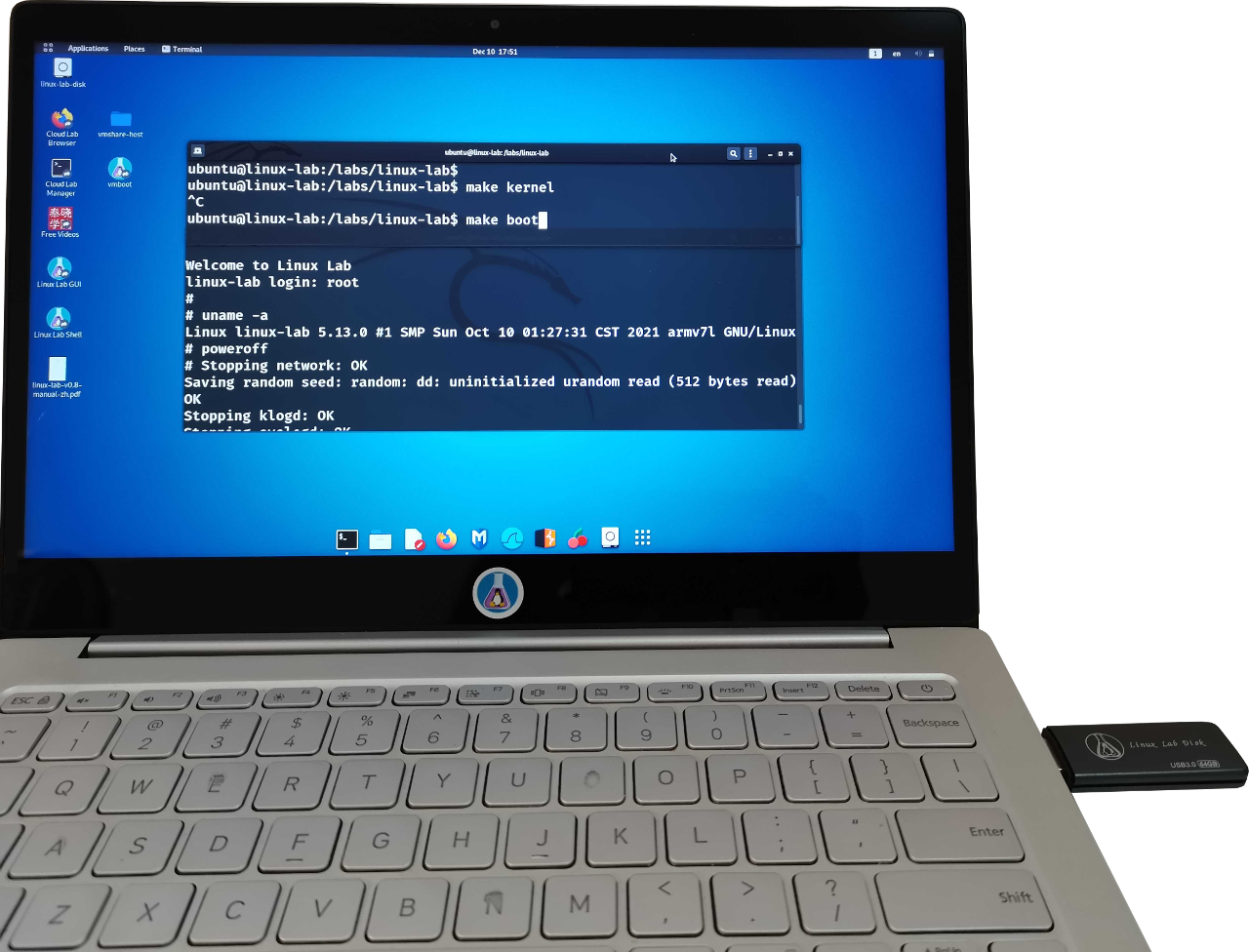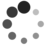[c-faq] 3.16-左值和右值

by Pingbo Wen of TinyLab.org 2013/08/11

An object is a region of storage that can be examined and stored into. An lvalue is an expression that refers to such an object.

L = R;

int x;
x=1;

1=x;

(m+1)=n;

int m,*p;
p=&m;
&m=p; //error

((condition) ? a : b) = complicated_expression; //error
*((condition) ? &a : &b) = complicated_expression; //correct

char *p;
((int *)p)++;

p = (char *)((int *)p + 1);
• 参考资料